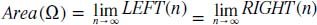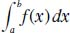NextPrevious

# What is the definite integral?

In actuality, the area shown on page 233 is actually determined using limits. In the function f(x), as n gets larger, the numbers determined by left (n) and right (n) will get closer and closer to the area Ω. This is seen as the following notation:Thus, in the calculus terms, the area of the above graphic region is called the definite integral (also said as “the integral”) of f(x) from a to b, and is denoted by the following notation:The variable x can be replaced with any other variable. In other words, if the limits of integration (a and b) are specified, it is called a definite integral, and it can be interpreted as an area or a generalization of an area.

Close

This is a web preview of the "The Handy Math Answer Book" app. Many features only work on your mobile device. If you like what you see, we hope you will consider buying. Get the App